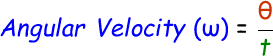# Angular Velocity Calculator

Angular velocity is a vector quantity which helps us to calculate the number of rotation a spinning object undergoes for the given amount of time period. The angular velocity is also known as rotational velocity.

We can calculate the angular velocity with the help of this below formula:where,
ω = Angular velocity [radians/sec]
θ = Angular displacement [radians]
t = Time taken [sec]

In the below online angular velocity calculator, enter the angular displacement and time taken in the input boxes and click calculate to find the angular velocity.

 Angular Displacement (θ): [radians] Time Taken (t): [seconds] Angular Velocity (ω): [radians/sec]

Latest Calculator Release

Average Acceleration Calculator

Average acceleration is the object's change in speed for a specific given time period. ...

Free Fall Calculator

When an object falls into the ground due to planet's own gravitational force is known a...

Torque Calculator

Torque is nothing but a rotational force. In other words, the amount of force applied t...

Average Force Calculator

Average force can be explained as the amount of force exerted by the body moving at giv...

Angular Displacement Calculator

Angular displacement is the angle at which an object moves on a circular path. It is de...